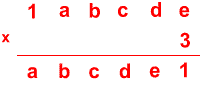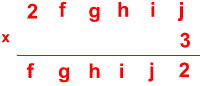#### You may also like### Cubes

How many faces can you see when you arrange these three cubes in different ways?### Pebbles

Place four pebbles on the sand in the form of a square. Keep adding as few pebbles as necessary to double the area. How many extra pebbles are added each time?### Bracelets

Investigate the different shaped bracelets you could make from 18 different spherical beads. How do they compare if you use 24 beads?

# Trebling

##### Age 7 to 11 Challenge Level:Can you replace the letters with numbers?
Is there only one solution in each case?

Once you've had a chance to think about it, click below to see how two different pupils began working on the task.

Here is Abdullah's work:

"For each problem I first looked to find a number that would make the ones column accurate, then I substituted the number for the answer in the tens column and then continued the process until the calculation was complete."

Joshua wrote:

"I wrote out single digit multiples of three up to 9 because each letter was one digit. I noticed that the numbers 1 to 9 only appeared once in the ones column of the answers. I looked at the question and realised that 3 x e had to be 21 because it was the only answer ending in 1. This meant that e had to be 7.

I carried the 2 and took it from 7 (the other e) and got 5. So d x 3 had to end in 5 which meant d had to be 5 because 5 x 3 = 15. I then repeated the process."

Can you take each of these starting ideas and develop it into a solution?